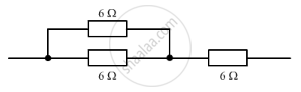Share

# The Diagram Below Shows Part of a Circuit: - CBSE Class 10 - Science

ConceptResistance of a System of Resistors - Resistors in Series

#### Question

The diagram below shows part of a circuit:If this arrangement of three resistors was to be replaced by a single resistor, its resistance should be:

(a) 9 Ω
(b) 4 Ω
(c) 6 Ω
(d) 18 Ω

#### Solution

(a) 9 Î
The two resistors of 6 Î are connected in parallel with each other. So, their net resistance 3 Î is connected in series with a resistance of 6 Î. So, the net resistance of the complete arrangement is 9 Ω.

Is there an error in this question or solution?

#### APPEARS IN

Solution The Diagram Below Shows Part of a Circuit: Concept: Resistance of a System of Resistors - Resistors in Series.
S